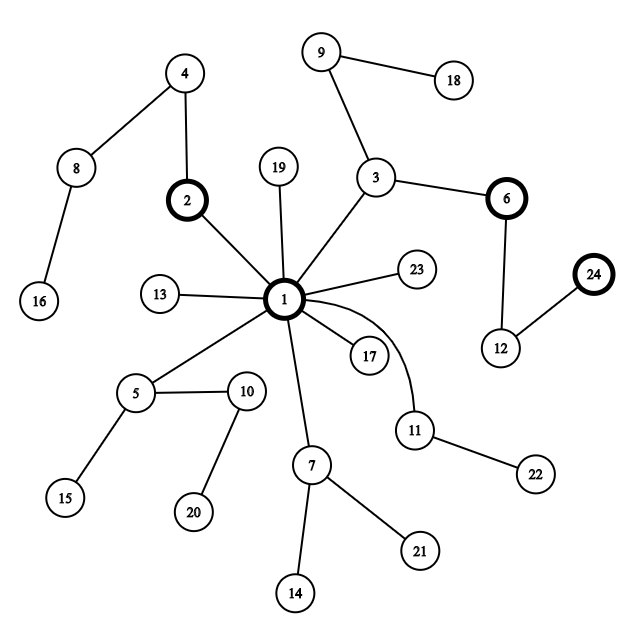# [CF1292D]Chaotic V.$1*3=3,3*2=6,6*2=12,12*2=24$

1. 如果$$p'$$能使答案更小，则$$p=p'$$，并重新统计$$p$$的各个子树关键点的数量；
2. 否则$$p$$就是使答案最小的点，退出循环。

posted @ 2020-01-20 13:07  青君  阅读(405)  评论(0编辑  收藏  举报